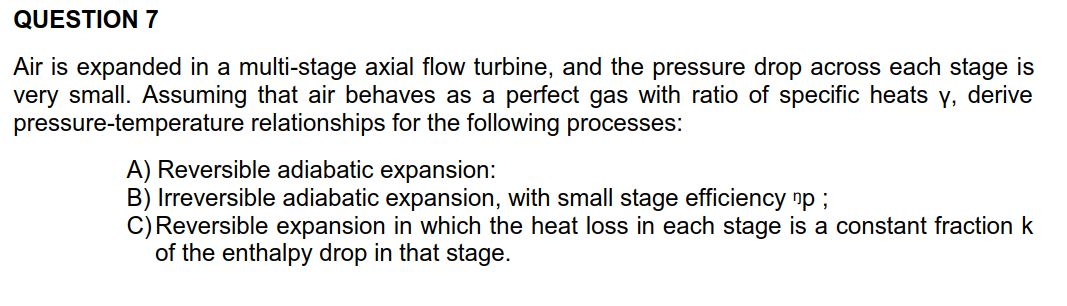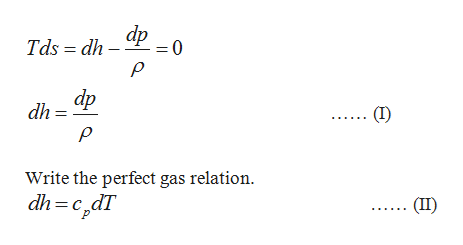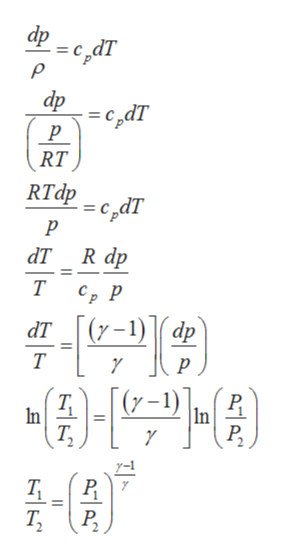# QUESTION 7multi-stage axial flow turbine, and the pressure dropAir is expanded in avery small. Assuming that air behaves as apressure-temperature relationships for the following processes:across each stage isperfect gas with ratio of specific heats y, deriveA) Reversible adiabatic expansion:B) Irreversible adiabatic expansion, with small stage efficiency np;C) Reversible expansion in which the heat loss in each stage is a constant fraction kof the enthalpy drop in that stage.

Question
201 viewshelp_outlineImage TranscriptioncloseQUESTION 7 multi-stage axial flow turbine, and the pressure drop Air is expanded in a very small. Assuming that air behaves as a pressure-temperature relationships for the following processes: across each stage is perfect gas with ratio of specific heats y, derive A) Reversible adiabatic expansion: B) Irreversible adiabatic expansion, with small stage efficiency np; C) Reversible expansion in which the heat loss in each stage is a constant fraction k of the enthalpy drop in that stage. fullscreen
check_circle

Step 1

Write the basic equation of thermodynamics with ds = 0.help_outlineImage Transcriptionclosedp -= 0 Tds dh P dp dh = (I) Write the perfect gas relation dh=c,dT (II) fullscreen
Step 2

From Equations (I) and (II),help_outlineImage Transcriptioncloseфр ес ат dp с,dт р RT RTdр с, dT р аT R dp Т СР р dт [(у-1)1( ар Т р 1-4) In Р. Т, In Т, Эt0- Р. Т, II fullscreen
Step 3

Write the basic equation of thermodynamics with Tds = 0 for a c...

### Want to see the full answer?

See Solution

#### Want to see this answer and more?

Solutions are written by subject experts who are available 24/7. Questions are typically answered within 1 hour.*

See Solution
*Response times may vary by subject and question.
Tagged in

### Mechanical Engineering